# Mathematics

Topic | v1 | created by semantic-scholar-bot |
Description
unavailable

Source unavailable
##### Relations

In mathematics, homology is a general way of associating a sequence of algebraic objects, such as abe...Category theory formalizes mathematical structure and its concepts in terms of a labeled directed gra...A computer algebra system (CAS) or symbolic algebra system (SAS) is any mathematical software with th...Geometry (from the Ancient Greek: γεωμετρία; geo- "earth", -metron "measurement") is, with arithmetic...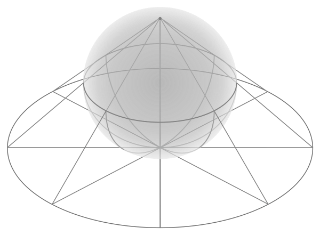In mathematics, Euler's identity (also known as Euler's equation) is the equality e to the π i = -1,...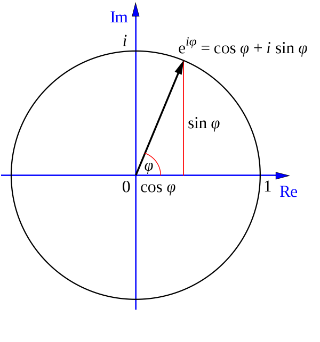Number theory (or arithmetic or higher arithmetic in older usage) is a branch of pure mathematics dev...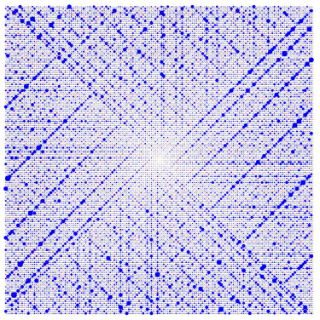Statistics is the discipline that concerns the collection, organization, analysis, interpretation, an...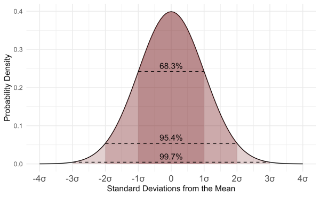In mathematics, the axiom of choice, or AC, is an axiom of set theory equivalent to the statement tha...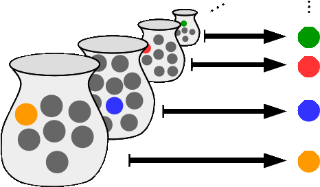Enter the world of the Mathologer for really accessible explanations of hard and beautiful math(s)....##### Resources

A Programmer's Introduction to Mathematics uses your familiarity with ideas from programming and soft...Cognicull is a learning site that pursues "ease of understanding" and "comfort". It is designed so th...Learn to understand math, not memorize it. Enjoy clear, intuitive lessons about imaginary numbers, ex...Welcome to my math notes site. Contained in this site are the notes (free and downloadable) that I us...